Common Limits on the AP Calc Exam

As you prepare for the AP Calculus exam, it’s important to know what you’re getting into. Hopefully you’ve already taken a look at the list of topics, but this article is about one topic in particular: limits. Read on to familiarize yourself with the common limits that you’ll encounter on the test!The limit of a function at a particular point is the y-value that the function approaches near that point.

What is a Limit?

The concept of limits is fundamental to bridging the gap between elementary mathematics (arithmetic, geometry, algebra, trigonometry, etc.) and calculus. In fact limits provide the theoretic background on which the main tool of calculus, the derivative, is built.

So it’s no surprise that limits show up on both the AB and BC versions of the AP Calculus exam.

We won’t take time in this short article to review the formal definition of limit. Instead, you can take a look at a number of helpful resources to review the concept.

Common Limits on the Exam

Now let’s get to some practice problems that highlight common limits on the AP Calculus exams.

1.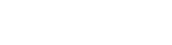2.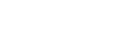3.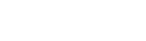4.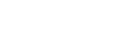5.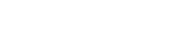6.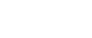7.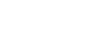8.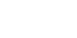1. Use the plug-in principle.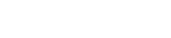2. Factor, cancel, then plug in.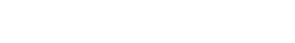3. This one is easier than it appears. Because the variable is heading toward infinity, all we have to do is a highest-order term analysis. Now since the highest order term on top ( 3x2 ) has lower degree than the one on the bottom ( x3 ), the limit must be 0.
4. Again this is a limit as x → ∞, so use highest-order term analysis. But this time, the top has a higher degree than the bottom. Isolate the highest degree terms on top and bottom:

( x7 ) / ( x5 ) = x2

Therefore, as x → ∞, our function behaves like x2. So in the limit, the values simply grow without bound. The limit is ∞.

5. Rounding out this trio of related examples, we have another limit in which x approaches one of the two infinities. Using highest-order term analysis this time, we see that the degree of the top is the same as the degree of the bottom. Let’s simplify.

( 7x4 ) / ( 5x4 ) = 7/5

This implies that as x → -∞, the function values get closer to the constant 7/5. That’s our limiting value!

6. Notice that plugging in x = 2 results in nonzero/zero, so we know the answer will be infinite.

When x is close to 2, but to the right of it, the top will be negative while the bottom is positive. Therefore, the limit is -∞.

7. Absolute values must be treated with care. When x is less than -1, the quantity (x + 1) is negative. So you must switch the sign to get rid of the absolute value bars.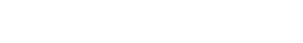8. You can either adjust the limit so that it has the form (sin θ)/θ, or just use l’Hospital’s Rule. The solution here uses the latter.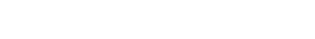Conclusion

Now that you’ve seen a number of common limits, you can be that much more confident going into the test. But keep in mind, there are many variations possible on these limit problems, so be on your guard!

By the way, if you want to review what else is on the exams, check out the following links.

Improve your SAT or ACT score, guaranteed. Start your 1 Week Free Trial of Magoosh SAT Prep or your 1 Week Free Trial of Magoosh ACT Prep today!Author

•Shaun earned his Ph. D. in mathematics from The Ohio State University in 2008 (Go Bucks!!). He received his BA in Mathematics with a minor in computer science from Oberlin College in 2002. In addition, Shaun earned a B. Mus. from the Oberlin Conservatory in the same year, with a major in music composition. Shaun still loves music -- almost as much as math! -- and he (thinks he) can play piano, guitar, and bass. Shaun has taught and tutored students in mathematics for about a decade, and hopes his experience can help you to succeed!